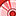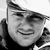Announcements
You can succeed best and quickest by helping others to succeed. Join the conversation.
cancel
Showing results for
Did you mean:Contributor III

## Set analysis greater than with multiple date fields used in set analysis

Hi,

I am trying to get data before 2019-05-31 with my YTD formula.

My current YTD formula works and it is the following:

sum({\$<Category={'Brand'},Device={'Desktop'},WeekStart=,MonthYear=,Year={"\$(=Max(Year))"},Date={"<=\$(=max(Date))"}>}Clicks)

Now when i want to extract before 2019-05-31 the set analysis doesn't work anymore. However, when I do greater than it works. The highlight in red is what i added to the set analysis.

sum({\$<Category={'Brand'},Device={'Desktop'},WeekStart=,MonthYear=,Year={"\$(=Max(Year))"},Date={"<=\$(=max(Date))"}, Date={">=\$(=Only(2019-05-31))"}>}Clicks)

My theory is that it is not work because I am using max(date) before therefore it wont work.

The reason i want to get before May 31st is because after that date the estimated conversion % changed.

Can someone help me to get the current YTD to date for before May 31st. ?

Labels (3)

• ### ytd

1 Solution

Accepted SolutionsContributor III

I think you are on the right track to use only one field selection for Date in set expression.  You won't need both

Date={"<=\$(=max(Date))"} and Date={">=\$(=Only(2019-05-31))"}

The Date part like this should work - Date={"< =dateformatted"}>}.  Date formats can be tricky in Qlik.

Can you try this expression?

sum({\$<Category={'Brand'},Device={'Desktop'},WeekStart=,MonthYear=,Year={"\$(=Max(Year))"}, Date={"<2019-05-31"}>} Clicks)

3 RepliesContributor III

I think you are on the right track to use only one field selection for Date in set expression.  You won't need both

Date={"<=\$(=max(Date))"} and Date={">=\$(=Only(2019-05-31))"}

The Date part like this should work - Date={"< =dateformatted"}>}.  Date formats can be tricky in Qlik.

Can you try this expression?

sum({\$<Category={'Brand'},Device={'Desktop'},WeekStart=,MonthYear=,Year={"\$(=Max(Year))"}, Date={"<2019-05-31"}>} Clicks)MVP

You need to make sure that your application date format is YYYY-MM-DD AMsnd you need to define your input as a string like this:

Date={">=\$(=Only('2019-05-31'))"}Contributor III
Author

Hi @john_obrien ,